# Birding Despite Disability

## The Physics of Roof Prisms and Phase Coatings, Simplified: Part II

#### Phase shifts and interference

The previous post introduced the topic of roof prism performance loss resulting from phase shifts that accompany total internal reflection (TIR). These shifts are endemic to TIR and to any device that employs it, including not only roof but Porro prisms. But in the latter case, the shifts have no deleterious effect on the image. The unique roof prism construction is therefore a necessary part of the problem. Only by tracing the paths that light takes through these devices can one see how phase shifts and prism geometry can together conspire to degrade image quality.

Porro prisms are not affected because all of the passing light undergoes the same net shift in phase. This does not present am issue as it has no detectable effect. It is by only by shifting some of the light, relative to the rest , in which something noticeable happens. This is a consequence of linear superposition, i.e., the fact that waves add together. Take two identical waves with the same amplitude A (corresponding, say, to the vertical height of the string) and add them, and you’ll get a result with amplitude 2A; this is constructive interference. But phase shift one wave relative to the other before you sum them and you’ll get a wave with amplitude less than 2A. For the case of a half-wavelength shift, the amplitude adds to zero and the waves cancel out in perfect destructive interference. (This will be animated in figure 9 below.)

When light enters a roof prism, it is cleaved into two portions that follow different routes before being recombined, and the two routes may accrue phase changes in different ways. Some amount of destructive interference can result from this, reducing the overall intensity of the transmitted light and causing a loss in resolution, which we will get to in the following post. Our goal here is only to trace through the passage of light and understand just how convoluted the entire process is. We will do this with a series of diagrams and animations that are schematic in nature; attempting to explicitly depict all of the sinusoidal plane waves careening about inside the prism would be a fool’s errand. What will hopefully become clear is how the conditions are ripe for light to undergo destructive interference, and why so much of the math in the technical treatment is dedicated to tracking the convoluted process of multiple reflections.

#### The subtleties of roof prism geometry

That the two paths through the prism could affect light differently is not obvious. In fact, the symmetry of the device would seem to preclude the idea at first glance. For example, as we begin to think about the problem, we might sketch a simple diagram such as that in figure 1(a). Here, light falling perpendicularly onto the two reflecting surfaces would seem to be treated equally since all four incidence angles are the same. We might conclude that we have oversimplified this, since light might come in from off-axis as well, as shown in figure 1(b). But when tracing through this geometry, we’ll see that both sides undergo reflections at the same two angles, just in different order, which seems like it shouldn’t matter.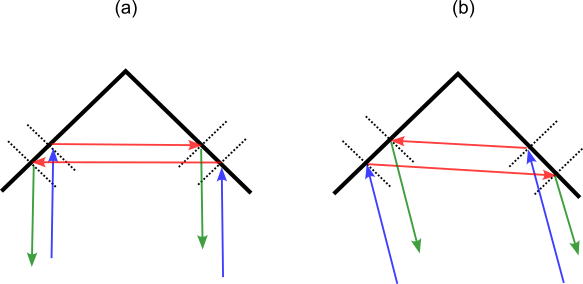Figure 1. Reflections of parallel rays falling onto either side of a roof prism. In both cases, each beam undergoes the same reflections.

To see what is breaking the symmetry requires looking more closely and recognizing that we’ll have to deal with the polarization of the light, which is to say, the electric field orientation. Each photon, or individual unit of light, amounts to a localized wave in the electric field; a wave that must be oriented at some angle perpendicular to the direction that the light travels. When we have light as a whole that is unpolarized, as is very often the case, it is only because it has no net polarization on average. It is still ultimately composed of photons that are each polarized. So we need to reckon with polarized light before we can do anything with the unpolarized case.

Suppose we start with a laser source, where the beam will feature a single wavelength and will be polarized. We split this into two parallel rays that enter a roof prism oriented such that the roof line makes a 45 degree angle to the light, as shown in figure 2(a). We will suppose that the light is polarized vertically, with the electric field pointing down as it approaches the surfaces, as shown by the purple arrows in figure 2(b). The field is of course oscillating, and pointing up and down as a function of position and time. However it is going to make life much simpler if we simply specify one direction. We will refer to the two sides, and the two beams, as the left and right portions, corresponding to first surface struck from the perspective of the light source, as shown in figure 2(c). This point of view will be referred to simply as our reference frame. The vertical direction corresponds to the z-axis, the rightward horizontal direction is the x-axis, and the propagation direction of the initial light is into the screen (or paper), along the y-axis.Figure 2. Geometries for ray tracing through the prism. Diagrams (a) and (b) depict how a single incident beam with vertical polarization is split into two before entering the prism. In (c) we are viewing the prism from the perspective of the light source, with the incident ray moving in the y-direction, into the screen.

If the prism were standing up straight so that the roof line was perpendicular to the light (imagine rotating the prism in 2(a) clockwise by 45 degrees), then the two rays would clearly strike their respective sides at 45 degree angles, relative to a “normal” or imaginary perpendicular line that sticks out of each face, as in figure 1(a). But with the prism itself at 45 degrees, the angle of first incidence (where blue arrows meet red) must be even steeper: it is 60 degrees relative to the normal direction. Upon reflection, the light moves off at 60 degrees, and will then strike the other surface (where red arrows meet green) at the same angle. Meanwhile the other ray takes a route that is a mirror image of this path. Attempting to depict all of this using two dimensional images is not easy, so we will resort to animations that change perspective in order to help visualize what is going on. Figure 3 shows the two interior sides of the roof and the two incoming rays in blue, their first reflections in red, and the exiting rays in green. The total deviation is 90 degrees for both, and they enter parallel and leave parallel. The purple arrows indicate the orientation of the electric field for the incoming light.Figure 3. Two parallel, incident rays (blue) within a roof prism are reflected once (red) such that they cross, and again (green) such that they leave parallel and at 90 degrees to the incoming light.

#### Tracing the passage of light

Let’s step back and recall what our task is now that we have a feel for the geometry. In the previous post, we saw that the phase shifts were dependent on the incidence angle and the polarization of the incoming light relative to the surface. The incidence angles are easy: all four reflections will all occur at 60 degrees relative to surface perpendiculars. It is the matter of polarization which is going to make a mess of this situation. Although we have chosen a simple starting condition, with vertical polarization in the frame of reference shown in figure 2(c), the perspective from the point-of-view of the surfaces is quite different (and far more important). The polarization arrows in figure 3 point neither in the s- nor p-polarization directions, but rather contain components in these directions. We will need to find these before we can talk about phase shifts. Because we are going to be breaking up these vectors into smaller components, we need to also be aware that their lengths correspond to the strength of the electric field, which determines the intensity of the light, which will be proportional to the square of the field.

In figure 4, we depict the incidence of the light schematically, by showing the polarization vectors approaching the prism surfaces. Each of these vectors is then resolved into two components, which correspond to the s- and p-polarization directions for the two surfaces. Note that while the original purple arrows were identical and parallel, none of the components are. The broken symmetry is apparent now. Keep in mind that nothing about the incident electric field has been changed; rather, we have two different frames of reference in which we must to describe it.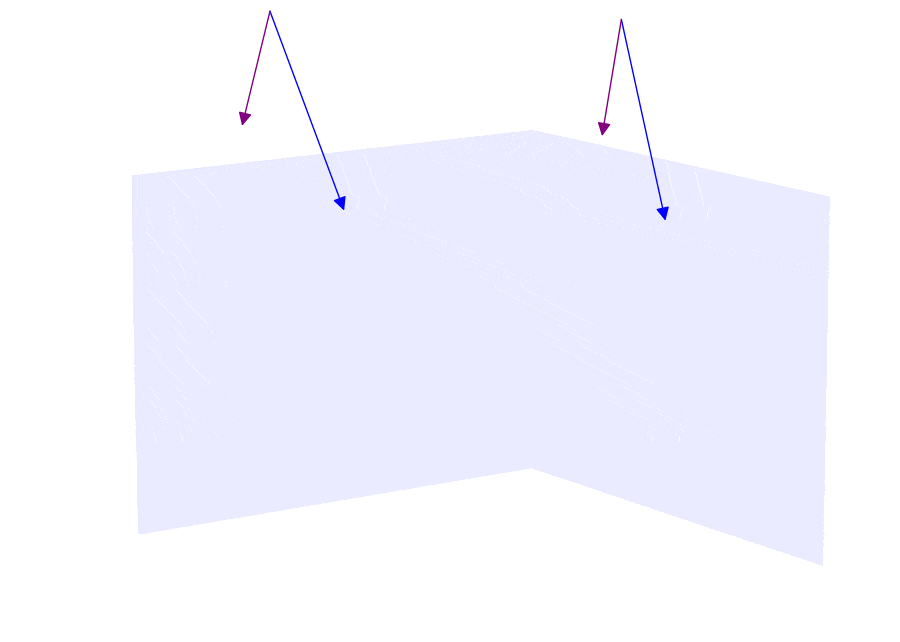Figure 4. Incident light approaches the prism with vertical polarization. In order to determine how it will be reflected, we need to resolve the electric field (purple) into components that are in the planes of the surfaces (s-polarization) and in the plane of incidence (p-polarization). Here, the longer of each pair corresponds to the s-direction.

When we resolved the electric field into these components, they are shown “attached” to the same point along the blue arrows, which indicate the direction of the propagation. This is a useful way to show the phase relationship of these two components – here they are perfectly in phase as they arrive at the surfaces. The purple arrows correspond to the location of the maximum field, which is of course changing in a sinusoidal manner in both time and space. Compare diagrams (a) and (b) in figure 5 to see how the components in figure 4 represent this phase information.Figure 5. Diagrams (a) and (b) show linearly polarized light resolved into p- and s-components. Diagrams (c) and (d) show the result of reflection with different phase shifts affecting the two components.

Upon reflection, we know that the p-component will acquire a larger phase shift than the s-component. Diagrams (c) and (d) in figure 5 show how these waves will then become staggered and how our simplified representation will capture this. (When this happens to light, the result is elliptical polarization, which means that the electric field vector will not oscillate back and forth, but will trace out the shape of an ellipse. While it is tempting to create some animations and digress on this, visualizing the elliptical polarization isn’t critical to understanding why the performance degrades.) With this in mind, we are ready for our next animation, in figure 6, which attempts to show what happens upon reflection. The p-components, which can be seen to lie in a plane defined by the red and blue arrows, precede the s-components, which are always parallel to their respective surfaces.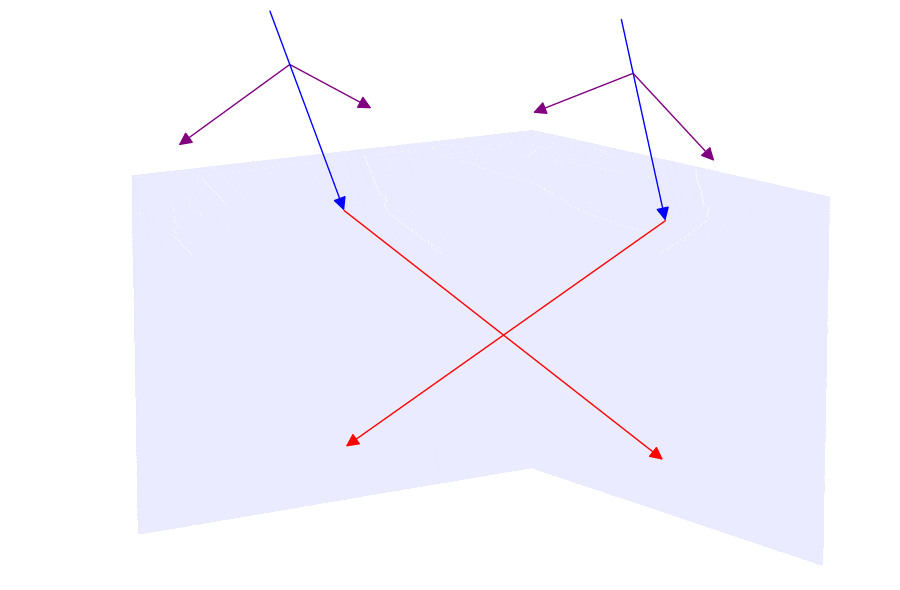Figure 6. Animation that depicts the phase shifts upon reflection for incoming, linearly polarized light. The phase shift upon reflection is captured by one component leading the other.

The p-vectors are seen to shift as they “go around the corner.” But they are in fact merely staying in the same plane: imagine holding a sheet of paper such that both the red and blue vectors for a given path lie on it. The p-component always remains in this plane, pointing perpendicular to the propagation direction. The lengths of all these vectors have not changed, but the difference in phase is about 11% of a wavelength, with the p- leading the s-component.

It would be convenient if these shifted components came out of their first reflections such that they were already in the s- and p-directions corresponding to the next set of reflections. Alas, they are not. Each of the four polarization vectors from figure 6 must now be resolved into new components prior to the upcoming reflections. We will perform the same kind of step as we did in figure 4, but now with four vectors being resolved into eight, as animated in figure 7.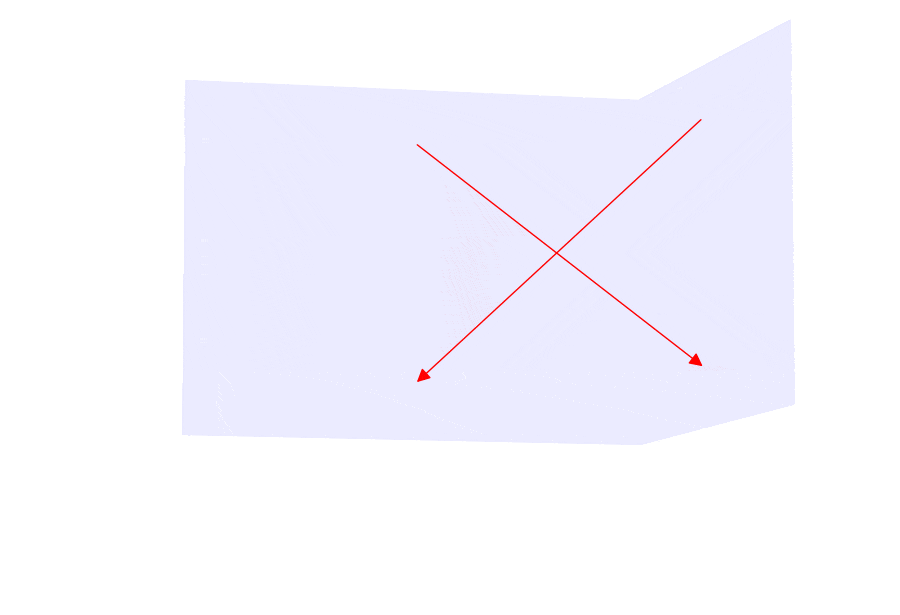Figure 7. The polarization vectors that were shifted in phase after the first reflection must now be resolved into s- and p-components corresponding to the next surfaces.

The bookkeeping needed to track all this is obviously going to get involved, because we need to take components of the components. (This is why the approach in the technical article is so much more attractive than doing this “by hand.” The methods used there take care of all of these components in a compact and elegant manner.)

Before looking at the next animation, it should be clear what we expect to see. Light that was p-polarized after the first reflection is now resolved into both s- and p-components for the second reflection, which will cause them to get shifted. The s-components from the first reflection, following behind, will also get split. As the rays finally come out of the prism, each one can be seen as consisting of four components. This is shown in figure 8. Again, there is no change to the vector lengths (so no change to the electric field strengths that they represent), but new phase shifts will need to be accounted for. We now will have the leading vector trailed by the next two, and a last one an equal distance back.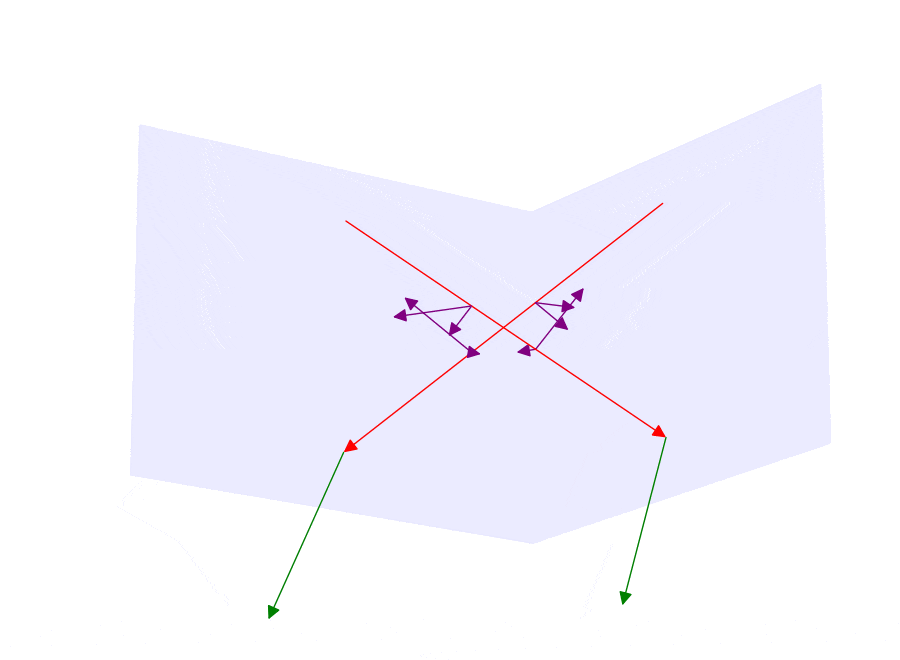Figure 8. After the second reflection, we have multiple components and phases shifts to reckon with.

Finally, with all the reflections completed, we will have to add together everything that was taken apart. We first note that for each path, there are two pairs of electric field vectors which are are parallel (or anti-parallel) to one another, but which differ in phase. To add these up we must keep in mind that we are not simply adding vectors, but sine waves that are shifted relative to one another (remember figure 5). The result of doing so produces another sine wave with a new amplitude and phase. Examples of adding shifted sine waves are shown in figure 9, for the cases of equal and unequal amplitudes. The waves to be added are in red and blue in the top row, while the bottom row curves in black show the results from summing them together. On the left side we have the special case of equal amplitudes, and we see the full range of constructive and destructive interference. But when the amplitudes are unequal, as on the right side, the summation is more involved, and we will obtain a sine wave with an amplitude dependent upon the relative phase. The phase of this resultant wave will also be affected. No matter what, the amplitude of the final wave can never be any larger than the sum of the two starting amplitudes.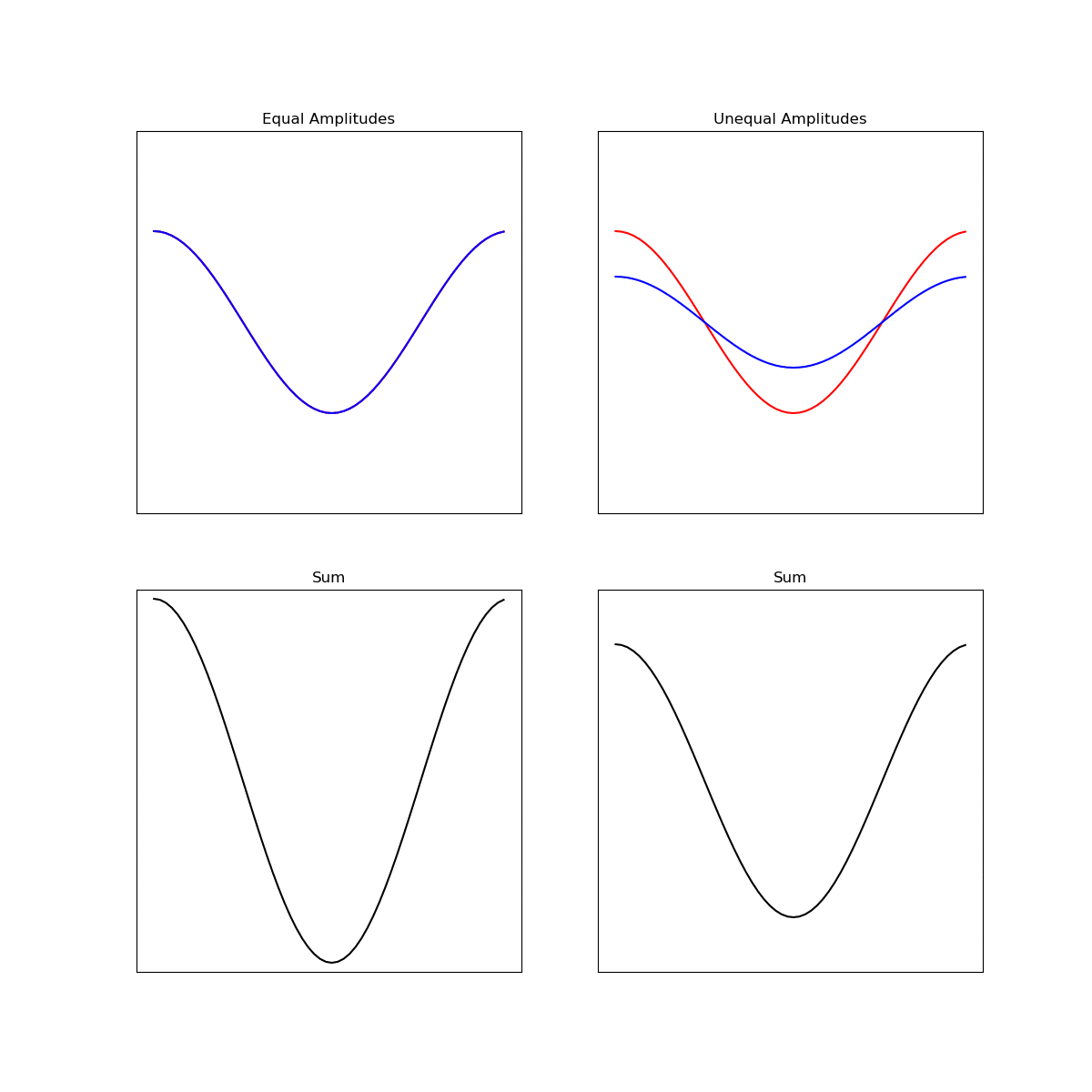Figure 9. The superposition of sine waves that are phase shifted. On the left we have two waves with equal amplitude, so when they are out of phase, the interference is completely destructive. On the right side we have unequal amplitudes. In this case the amplitude is usually less than the sum of the two but is never zero. The amplitude and phase of the sum both depend on the phase offset.

For each path, we will combine the two s-components and the two p-components based on the addition rule illustrated by figure 9. This will leave us with two set of shifted components for each path. The last step will be to add up these results, but this is not trivial either, because none of the vectors are parallel to one another. We will need to express the vectors for one of these paths in the coordinate system of the other path before we can sum them. This means we will be adding staggered sine waves again, and there will be another opportunity for some phase interference.

It should be pointed out that if there were no phase shift upon reflection, then everything would add back together perfectly. The great mess made by taking components of components will be perfectly reversed when they are all added back together. But the existence of the phase shifts throws a wrench into it all, providing an opportunity for destructive interference to get in and attenuate the total amount of light that escapes.

Keep in mind that as complicated as this all is, we have only looked at the simple case of a single polarization direction – and a symmetrical one that was relatively easy to track. If we want to consider unpolarized light, we need to repeat this for all other polarization orientations and add up the results. Moreover, in this example we are only looking at coherent light, i.e., light that comes in with the same phase. But if the light source contains a variety of phases distributed in a certain way, we will need to repeat these calculations for each incoming phase as well. That is exactly what we need to do to determine the effect on resolution. Luckily, integral calculus makes such computations straightforward, but formulating the problem correctly takes much care. The bulk of the technical article involves just setting up the integrations, and the calculus that comes on the heels of that is actually rather trivial.

In the next post we will look at the image deterioration that comes from combining all of these various phase shifted components. We’ll also look at how phase coatings can mitigate it.